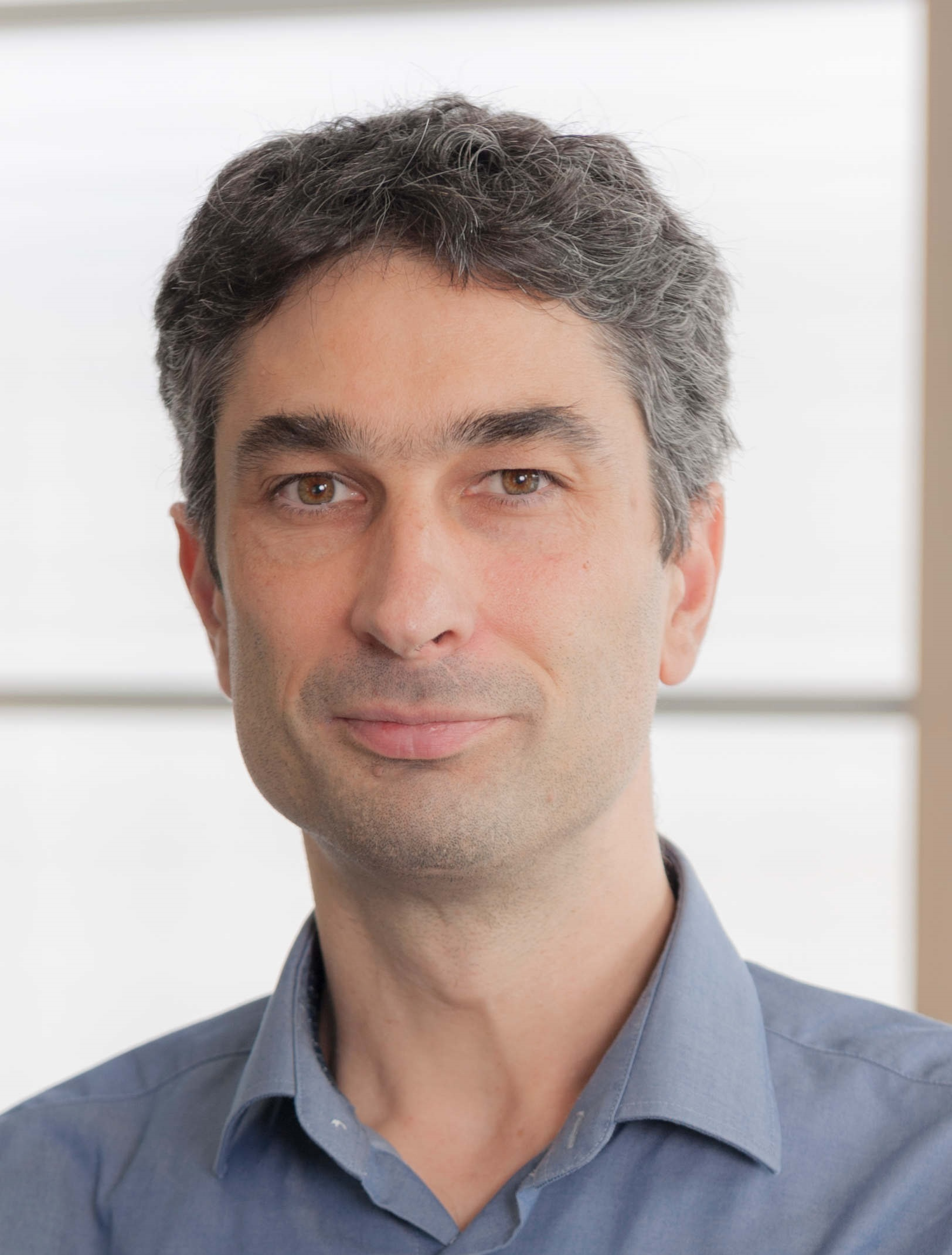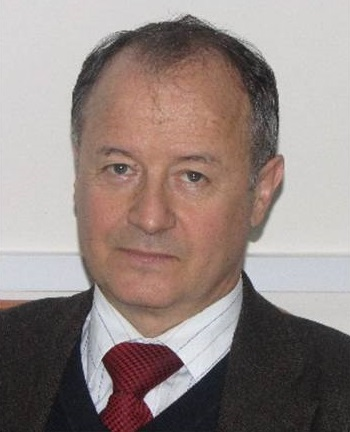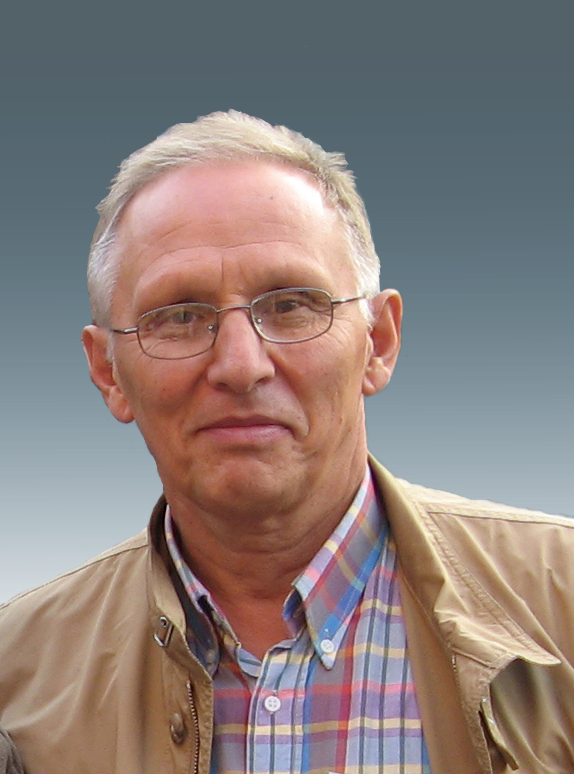#INTERNATIONAL CONFERENCE MATHEMATICAL OPTIMIZATION THEORY AND OPERATIONS RESEARCH (MOTOR 2021)

5-10 July 2021
Asia/Irkutsk timezone

## Invited Speakers

Invited SpeakersDr. Christian Blum

Artificial Intelligence Research Institute (IIIA-CSIC), Barcelona (Spain)
Senior Research Scientist

On the Design of Matheuristics that make Use of Learning

Approximate techniques for solving combinatorial optimization problems, such as metaheuristics, often make use of learning. Examples include evolutionary algorithms and ant colony optimization. On the other side, matheuristics - that is, heuristic techniques making use of mathematical programming - rarely include a learning component. Most variants of large neighbourhood search, for instance, do not take profit from learning. In this talk I will present examples of our recent work in which we design matheuristics that make successful use of learning, considering both positive and negative feedback.Prof. Emilio Carrizosa

Head of the Institute of Mathematics of the University of Seville (Spain)
Professor of Statistics and Operations Research at the University of Seville
Past President of SEIO (the Spanish Society of Statistics and Operations Research)

Optimal Classification and Regression Trees

Classification and Regression Trees are very powerful Machine Learning tools. Their design expressed as an optimization problem enables us to obtain excellent accuracy performance, and, at the same time, to have some control on important issues such as sparsity, explainability or fairness. In this talk, some recent advances in the field and future research lines will be discussed.Prof. François Clautiaux

Professor of Operations Research of Bordeaux University (France)
President of ROADEF (French Operations Research Society)

Integer programming formulations based on exponentially large networks: algorithms and applications

The last ten years have seen much progress in the field of so-called extended formulations, which aims at reformulating effectively a problem/polyhedron with the help of (exponentially many) additional variables. In particular, network-flow formulations have received an increasing interest from the community. A considerable difficulty to overcome when dealing with such a formulation is to handle its size. In this talk we recall some key results concerning these formulations, and present several recent successful applications that have been obtained using innovative aggregation/disaggregation techniques.Prof. Andreas Griewank

Professor Emeritus of the Institute of Mathematics at the Humboldt University (Germany)
Member of the Smart Data Analysis Systems Group

Potential based Losses for Classification without Regularization

For classification models in neural networks the standard loss formulation is based on the softmax function. We consider losses based on other "potential" functions including the sparse-max, or hard-max formulation proposed by Martins et al. These alternatives entail a loss in smoothness, but yield sparsity structure that can be used to drastically reduce the computational effort of evaluating gradients and (generalized) Hessians of the resulting risk objective. For linear, one-layer models even variations of Newton's method become competitive, provided  the highly structured Hessian matrix is factored approximately in a suitable fashion. Moreover, under the assumption that for suitable weights the prediction function is strictly consistent with the training data, we can prove that the risk objective has a zero infimum, which is actually attained as minimum in the hard-max case. We numerically verify the theoretical results and algorithmic proposals on classical test problems including  MINIST, Fashion MNIST and CIFAR 10.Prof. Klaus Jansen

University of Kiel (Germany)

Integer Programming and Convolution, with Applications

Integer programs (IP) with m constraints are solvable in pseudo-polynomial time. We give a new algorithm based on the Steinitz Lemma and dynamic programming with a better pseudo-polynomial running time than previous results.

Moreover, we establish a strong connection to the problem (min,+) - convolution. (min,+) - convolution has a trivial quadratic time algorithm and it has been conjectured that this cannot be improved significantly.

Finally we show for the feasibility problem also a tight lower bound, which is based on the Strong Exponential Time Hypothesis (SETH), and give some applications for knapsack and scheduling problems. This is joint work with Lars Rohwedder.Prof. Sergey Kabanikhin

Corresponding Member of Russian Academy of Sciences
Novosibirsk State University (Russia)

Optimization and Inverse Problems

Inverse problems arise in many applications in science and engineering. The term “inverse problem” is generally understood as the problem of ﬁnding a speciﬁc physical property, or properties, of the medium under investigation, using indirect measurements. In general, an inverse problem aims at recovering the unknown parameters of a physical system which produces the observations and/or measurements. Such problems are usually ill-posed. This is often solved via two approaches: a Bayesian approach which computes a posterior distribution of the models given prior knowledge and the regularized data ﬁtting approach which chooses an optimal model by minimizing an objective taking into account both ﬁtness to data and prior knowledge. Optimization plays an important role in solving many inverse problems. Indeed, the task of inversion often either involves or is fully cast as a solution of an optimization problem. In this talk, we discuss current state-of-the-art optimization methods widely used in inverse problems. We then survey recent related advances in addressing similar challenges in problems faced by the machine learning community and discuss their potential advantages for solving inverse problems.Prof. Nenad Mladenovic

Khalifa University, Abu Dhabi (UAE)

Minimum sum of squares clustering for Big Data – heuristic approach

We first present a review of local search methods that are usually used to solve the minimum sum-of-square clustering (MSSC) problem. We then present some their combinations within Variable neighbourhood descent (VND) scheme. They combine k-means, h-means and j-means heuristics in a nested and sequential way. To show how these local searches can be implemented within a metaheuristic framework, we apply the VND heuristics in the local improvement step of variable neighbourhood search (VNS) procedure. Computational experiments are carried out which suggest that this new and simple application of VNS is comparable to the state of the art. Then we discuss some decomposition and aggregation strategies for solving MSSC problem with huge data sets. Following the recent Less is more approach, the data set is divided randomly into a few smaller subproblems and after solving, the centroids of each subproblem is chosen to represent its cluster for a new aggregation stage. Encouraging computational results on instances of several million entities are presented.Prof. Claudia Sagastizábal

IMECC - University of Campinas (Brazil)

Exploiting structure in nonsmooth optimization

In many optimization problems nonsmoothness appears in a structured manner. Composite structures are found in LASSO-type problems arising in machine-learning. Separable structures result from applying some decomposition technique to problems that cannot be solved directly. This context is frequent in stochastic programming, bilevel optimization, equilibrium problems.

The talk will give a panorama of techniques that have proven successful in exploiting structural properties that are somewhat hidden behind nonsmoothness. Throughout the presentation the emphasis is put on transmitting the main ideas and concepts, illustrating with examples the presented material.Prof. Mikhail Solodov

Institute for Pure and Applied Mathematics (Brazil)

State-of-the-art on rates of convergence and cost of iterations of augmented Lagrangian methods

We discuss state-of-the-art results on local convergence and rate of convergence of the classical augmented Lagrangian algorithm. The local primal-dual linear/superlinear convergence is obtained under the sole assumption that the dual starting point is close to a multiplier satisfying the second-order sufficient optimality condition. In fact, in the equality-constrained case, even the weaker noncriticality assumption is enough. In particular, no constraint qualifications of any kind are needed. Classical literature on the subject required the linear independence constraint qualification (in addition to other things). In addition to the most standard form of the augmented Lagrangian algorithm, the general lines of analysis apply also to its variant with partial penalization of the constraints, to the proximal-point version, and to the modification with smoothing of the max-function.

Moreover, we show that to compute suitable approximate solutions of augmented Lagrangian subproblems which ensure the superlinear convergence of the algorithm, it is enough to make just two Newtonian steps (i.e., solve two quadratic programs, or two linear systems in the equality-constrained case). The two quadratic programs are related to stabilized sequential quadratic programming, and to second-order corrections, respectively.Dr. Dmitri Shmelkin

Lead of Mathematical Modeling Laboratory at Moscow Research Center of Huawei (Russia)

Novel scenarios and old challenges: Discrete Optimization at various Huawei technologies

In my talk I will introduce several mathematical directions from the domain of Discrete Optimization and Operational Research and having importance in different technologies researched by Mathematical Modeling Laboratory at Huawei. There are no engineering prerequisites required, and my target is to show much opportunities to do mathematical research with an impact to technologies.

TutorialsProf. Alexander Strekalovsky

Matrosov Institute for System Dynamics and Control Theory (Russia)

A Local Search Scheme for the Inequality-Constrained Optimal Control Problem

This paper addresses the nonconvex optimal control (OC) problem with the cost functional and inequality constraint given by the functionals of Bolza. All the functions in the statement of the problem are state-DC, i.e. presented by a difference of the state-convex functions. Meanwhile, the control system is state-linear. Further, with the help of the Exact Penalization Theory we propose the state-DC form of the penalized cost functional and, using the linearization with respect to the basic nonconvexity of the penalized problem, we study the linearized OC problem. On this basis, we develop a general scheme of the special Local Search Method with a varying penalty parameter. Finally, we address the convergence of the proposed scheme.Prof. Alexander Krylatov

Saint-Petersburg State University (Russia)

Equilibrium Traffic Flow Assignment in a Multi-subnet Urban Road Network

Today urban road network of a modern city can include several subnets. Indeed, bus lanes form a transit subnet available only for public vehicles. Toll roads form a subnet, available only for drivers who ready to pay fees for passage. The common aim of developing such subnets is to provide better urban travel conditions for public vehicles and toll-paying drivers. The present paper is devoted to the equilibrium traffic ow assignment problem in a multi-subnet urban road network. We formulate this problem as a non-linear optimization program and prove that its solution corresponds to the equilibrium traffic assignment pattern in a multi-subnet road network. Moreover, we prove that obtained equilibrium traffic assignment pattern guarantees less or equal travel time for public vehicles and toll-paying drivers than experienced by all other vehicles. The findings of the paper contribute to the traffic theory and give fresh managerial insights for traffic engineers.